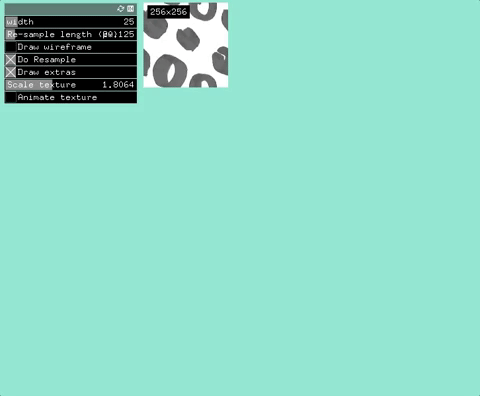# ofMesh LineStrips + textured

I normally render a line strip with opengl immediate calls or quad strips. When doing this you can set a color for each face. I am trying to use `ofMesh` to draw this using `OF_PRIMITIVE_TRIANGLE_STRIP`, I get a interpolated colors. Am I thinking about this problem wrong? How would I go about coloring each segment a separate color, like hard stripes.I want something like this:here is my attempt.

``````    ofSetColor(255);

ofMesh mesh;
mesh.setMode(OF_PRIMITIVE_TRIANGLE_STRIP);

if(points.size() > 1) {

ofVec2f a, b, v;
float width = 25;

for (int i = 0;  i < points.size(); i++){

if (i == 0) {
a = points[i];
b = points[i+1];
v = a - b;

} else {
a = points[i];
b = points[i - 1];
v = b - a;
}

ofVec2f p = v.perpendicular();

if (i%2==0) {
} else {
}
}

mesh.draw();

ofSetColor(255);
mesh.clearColors();
mesh.drawWireframe();
}

for (int i=0; i<points.size(); i++) {
ofDrawCircle(points[i], 3);
}``````

i think you need to repeat vertices in order to do “flat” colors per face, use triangles instead of strips

I got something, not sure that it is the most efficient but works! Here is the a git gist of the results.i can imagine you can use triangle stripe with some degenerate triangles to duplicate the vertices but i don’t think the vertex count would be much lower. just moving the mesh outside of the function into the instance and using a vbo mesh will probably optimize much more than saving a couple of vertices

Hey Todd,

Looked over the gist, you could also use polyline functions for getting the normals and tangents at indices. polyline.getNormalAtIndex(i) or polyline.getTangentAtIndex(i). I believe the result will be interpolated.

Cheers,
Nick

Holy Moly! `getNormalAtIndex` & `getNormalAtIndexInterpolated`

I update the gist - results are ok, starts to slow down at around 600 points. I think that a texture is the best approach I will try that out next.

1 Like

I made a texture line strip here. I had trouble making the texture fit properly somehow the math in there works. Any feed back would be great.2 Likes